# week3 .pdf

### File information

Original filename: week3.pdf

This PDF 1.5 document has been generated by LaTeX with hyperref package / pdfTeX-1.40.13, and has been sent on pdf-archive.com on 20/02/2016 at 16:22, from IP address 81.164.x.x. The current document download page has been viewed 599 times.
File size: 150 KB (5 pages).
Privacy: public file

week3.pdf (PDF, 150 KB)

### Document preview

Assignment Week 3: A Primal-Dual Algorithm for the k-median
Problem.
In this exercise, we propose to design a primal-dual algorithm for the k-median problem.
The k-median problem is defined as follows: given a complete bipartite graph G = (F ∪ C, E) where F
is the center set and C the client set and a metric distanceP
function c : E → R+ the goal is to find a
subset S ⊆ F containing at most k elements and such that v∈C minf ∈S c((v, f )) is minimized.
This problem is similar to the facility location problem, except that there is no cost for opening a
facility.
We want to design a primal-dual algorithm achieving a 6-approximation based on the primal dual
algorithm seen during the lectures.
The standard LP formulation, LP1, is as follows:
minimize
X

mini∈F xij c((i, j)).

(1)

j∈C

subject to
X

∀j ∈ C,

xij ≥ 1 ,

i∈F

∀j ∈ C, i ∈ F,

yi − xij ≥ 0 ,
X
yi ≤ k ,

(2)

i∈F

∀j ∈ C, i ∈ F ,

xij ≥ 0 ,

∀i ∈ F ,

yi ≥ 0 .

In order to have a LP, LP2, that is closer to the one we had for facility location, we will consider a
slightly different LP. For a given λ ≥ 0, we define the following LP.
P
P
minimize j∈C mini∈F xij c((i, j)) + λ( i∈F yi − k).
subject to
X

∀j ∈ C,

xij ≥ 1 ,

i∈F

∀j ∈ C, i ∈ F,

yi − xij ≥ 0 ,

∀j ∈ C, i ∈ F ,

xij ≥ 0 ,

∀i ∈ F ,

(3)

yi ≥ 0 .

The parameter λ is a way to penalize the LP for opening a lot of facilities. Indeed, we would like to
find a good value for λ so that an optimal solution for the LP will open at most k facilities.
Observe that any feasible solution for the linear programming relaxation of the k-median problem, LP1,
is also feasible for LP2.
Moreover, any feasible solution for LP2 is also a solution for LP1, assuming λ ≥ 0.
• Question 1: Give the dual of LP2, DUAL2.
The dual has variables αj , βij (i ∈ F, j ∈ C). The goal is:
maxj∈C αj − λk ,

1

(4)

subject to
∀j ∈ C, i ∈ F,

αj − βij ≤ c((i, j)) ,
X
βij ≤ λ ,

∀i ∈ F,

(5)

j∈C

∀j ∈ C ,

αj ≥ 0 ,

∀j ∈ C, i ∈ F ,

βij ≥ 0 .

Note the presence of the constant term −λk in the objective. Since the primal also has such a
constant term in the objective we have to add it to the dual to make the weak/strong duality
theorem work: the duality theorem works without the extra constant, then we just add the same
constant term to both the primal and dual objective.
We now would like to use the primal-dual algorithm seen during the lectures (the one that achieves
a 3-approximation) in which all the facility cost are equal to some λ ≥ 0.


P
P
P
Recall that in Lecture 8, we have seen that cluster C0 fiC0 + j∈C0 c((i, j)) ≤ 3 j∈C0 αj .


P
P
P
In fact, it is possible to show that cluster C0 3 fiC0 + j∈C0 c((i, j)) ≤ 3 j∈C0 αj .
• Question 2: Suppose that the fi are all equal to λ and that the algorithm opens a set S of facilities,
what can you deduce from the above formula?
P
P
We have cluster C0 3 fiC0 = cluster C0 3 λ = 3 λ|S| and thus
XX
X
3 λ|S| +
c((i, j)) ≤ 3
αj .
(6)
C0 j∈C0

j∈S

• Question 3: Suppose further that the set S contains exactly k facilities, what can you deduce from
the cost of the solution output by the algorithm and the value of the dual?
P P
From Q2 and the objective of LP2, cost(S) = C0 j∈C0 c((i, j)) + λ(|S| − k), we find

X
2 λ(|S| − k) + cost(S) ≤ 3 
αj − λk  = 3 val(α) ≤ 3 OPT ,
(7)
j∈S

and putting |S| = k

X
cost(S) ≤ 3 
αj − λk  = 3 val(α) ≤ 3 OPT

(8)

j∈S

We now want to find the value of λ that would lead the algorithm to open exactly k facilities.
In order to do so, we will do a bisection search and maintain a lower bound λ1 and an upper
bound λ2 on the value of the optimal λ.
P
P
We start with λ1 = 0 and λ2 = j∈C i∈F c((i, j)).
• Question 4: Prove that in the case of λ = λ1 , the algorithm opens at least k facilities and in the
case of λ = λ2 the algorithm opens less than k facilities.
If λ = λ1 = 0, the algorithm blocks for each client j the edge with minimum cij , i.e. the edge
to the closest facility, with αj = mini cij . It also blocks this closest facility. Assuming all edge
weights are different no client is connected with a minimal edge to two facilities (if there are equal
minimal edges we can arbitrarily chose one to be blocked). It follows that there are no clients at
distance 3 to a blocked facility and all blocked facilities are opened. So the opened facilities are
all the facilities which are closest to at least one edge. If there is no constraint on the number of
facilities, this is obviously the optimal solution. We assume the number of such facilities is bigger
than k, otherwise the problem is trivial.
2

P
If λ = λ2 , the first facility i to be blocked is the one for which j cij is minimal. At that point we
have αj ≥ (λ2 + mini cij ) /|C| ≥ cij for all j, all edges are blocked, and all clients are at distance
at most 3 of the facility. So only one facility is opened.
We start by running the algorithm for λ1 , which returns a set of facilities S1 . Then we run the
algorithm for λ2 and obtain a set of facilities S2 . If |S1 | &gt; k and |S2 | &lt; k, we run the algorithm
on the value λ = (λ1 + λ2 )/2.
The algorithm outputs a set S of facilities. If |S| &gt; k, we set λ1 = (λ1 + λ2 )/2, S1 = S and repeat.
Otherwise we set λ2 = (λ1 + λ2 )/2, S2 = S and repeat.
We repeat until we obtain a set S of k facilities or λ2 − λ1 is small enough.
In the later case, we explain how to combine S1 and S2 to obtain a solution with k facilities.
Let cmin be the smallest assignment cost greater than 0. We run the bisection search until we get
a set S of k facilities or until λ2 − λ1 ≤  cmin /(3|F |), for some fixed  &gt; 0.
If we have not terminated with a solution with exactly k facilities, the algorithm terminates with
solutions S1 and S2 and (corresponding) dual solutions (α1 , β 1 ) and (α2 , β 2 ) such that |S1 | &gt; k &gt;
|S2 |.
• Question 5: Use Question 3 to derive an inequality connecting the value of the solution induced
by the set S1 , cost(S1 ), and (α1 , β1 ) and λ1 . Derive a similar bound for the value of the solution
induced by S2 , cost(S2 ).
For simplicity let us define the cost without the Lagrange multiplier part
XX
cost0 (S) =
c((i, j)) = cost(S) − λ(|S| − k) .

(9)

C0 j∈C0

From Q3 we find then for S1
cost0 (S1 ) ≤ 3

X

αj1 − 3 λ|S1 | ,

(10)

αj2 − 3 λ|S2 | .

(11)

j

and S2
cost0 (S2 ) ≤ 3

X
j

Without loss of generality, we can assume that 0 &lt; cmin ≤ OPT , since if OPT = 0 then it is easy
to compute an optimal solution.
We now pick δ1 , δ2 &gt; 0 such that δ1 + δ2 = 1 and δ1 |S1 | + δ2 |S2 | = k.
˜ by letting α
We can then get a dual solution (˜
α, β)
˜ = δ1 α1 + δ2 α2 and β˜ = δ1 β1 + δ2 β2 .
˜ is feasible for the DUAL2 with facility costs λ2 since it is a convex combination
Note that (α
˜ , β)
of two feasible dual solutions. We can now prove the following lemma.
It states that the convex combination of the costs of the solutions induced by S1 and S2 must be
close to the cost of an optimal solution.
Lemma 1. δ1 cost(S1 ) + δ2 cost(S2 ) ≤ (3 + δ1 )OPT.
• Question
6: Using Question
5 and the fact that λ2 − λ1 ≤ cmin /(3|F |), prove that cost(S1 ) ≤
P

1
3
j∈C αj − λ2 |S1 | +  OPT.

3

We find using respectively Q5, |S1 | &gt; k, −λ1 ≤ cmin /(3|F |) − λ2 , cmin ≤ OPT and |S1 | ≤ |F |
X
cost0 (S1 ) ≤ 3
αj1 − 3 λ1 |S1 |
j

≤3

X

αj1 − 3 λ2 |S1 | +  cmin

j

|S1 |
|F |

(12)

X
≤3
αj1 − λ2  +  OPT .
j

• Question 7: Using a convex combination of cost(S2 ) and the inequality derived in Question 6,
conclude the proof of Lemma 1.
Combining Q5 and Q6 we find
δ1 cost0 (S1 ) + δ2 cost0 (S2 ) ≤ 3 δ1

X

αj1 + 3 δ2

j

X

αj2 − 3 (δ1 + δ2 ) λ2 + δ1  OPT

j

≤ 3 val(α
˜ ) + δ1  OPT

(13)

≤ (3 + δ1 ) OPT .
Let us now consider the original costs:
δ1 cost(S1 ) + δ2 cost(S2 ) = δ1 cost0 (S1 ) + δ2 cost0 (S2 ) + δ1 λ1 (|S1 | − k) + δ2 λ2 (|S2 | − k)
≤ δ1 cost0 (S1 ) + δ2 cost0 (S2 ) + δ1 λ1 (|S1 | − k) + δ2 λ1 (|S2 | − k)
cmin
(|S2 | − k)
+ δ2
3|F |
≤ 0,

(14)

where we used λ2 − λ1 ≤ cmin /(3|F |), δ1 |S1 | + δ2 |S2 | = k and |S2 | ≤ k. Lemma 1 follows.
We now conclude the analysis. We need to distinguish two cases, δ2 ≥ 1/2 and δ2 &lt; 1/2.
In the case of δ2 ≥ 1/2, we return the set S2 . Recall that |S2 | &lt; k and thus, S2 is feasible.
• Question 8: Assuming δ2 ≥ 1/2 and using Lemma 1, show that the set S2 is a solution of cost at
most 2(3 + )OPT.
From the lemma we find
cost(S2 ) ≤ 1/δ2 (δ1 cost(S1 ) + δ2 cost(S2 )) ≤ 1/δ2 (3 + δ1 )OPT ≤ 2(3 + δ1 )OPT .

(15)

We now assume that δ2 &lt; 1/2.
Then, for each facility i ∈ S2 , we open the closest facility h ∈ S1 ; that is, the facility h ∈ S1 . If
this doesn’t open |S2 | facilities of S1 because some facilities in S2 are close to the same facility
in S1 , we open some arbitrary facilities in S1 so that exactly |S2 | are opened. We then choose a
random subset of k − |S2 | of the |S1 | − |S2 | facilities of S1 remaining, and open these. Let S be
the resulting set of facilities opened.
We show that the expected cost of S is at most 2(3 + )OPT.
We give a bound on the expected cost of assigning a given client j to a facility opened by the
randomized algorithm.
Let us suppose that the facility f1 ∈ S1 is the open facility in S1 closest to j. This means that
c((f1 , j)) is the contribution of client j to the cost of S1 , c1j .
Let f2 ∈ S2 be the open facility in S2 that is the closest to j. Again, c((f2 , j)) is the contribution
of client j to the cost of S2 , c2j .
Recall that

k−|S2 |
|S1 |−|S2 |

= δ1 .
4

• Question 9: What is the probability that the randomized algorithm opens f1 ?
The algorithm opens k − |S2 | facilities of the remaining |S1 | − |S2 |, so the probability is δ1 =
k−|S2 |
|S1 |−|S2 | .
If f1 is open, then j is assigned to f1 . Otherwise, we assign j to the closest facility of S1 closest
to f2 . Let i be this facility. Recall that by the triangle inequality we have that c((i, j)) ≤
c((j, f2 )) + c((f2 , i)).
• Question 10: Give an upper bound on the distance of c((i, f2 )) based on the distance from
c((f1 , f2 ))
We have:
c((i, f2 )) ≤ c((f1 , f2 ))

(16)

because i is the closest facility to f2 of S1 and thus closer than f1 .
• Question 11: Show that c((i, j)) ≤ c1j + 2c2j .
Using the triangle identity and Q10 we find:
c((i, j)) ≤ c((j, f2 )) + c((i, f2 )) ≤ c((j, f2 )) + c((f1 , f2 )) ≤ c((j, f1 )) + 2 c((j, f2 )) = c1j + 2 c2j . (17)
• Question 12: Based on Questions 9 and 11, show that the expected cost for client j is at most
δ1 c1j + δ2 (c1j + 2c2j ).
The probability that f1 is opened is δ1 and the cost for client j is then c1j , in the other case, with
probability 1 − δ1 = δ2 , the cost is c((i, j)).
We find for the expected cost using Q11:
δ1 c1j + δ2 c2j ≤ δ1 c1j + δ2 (c1j + 2c2j ) .

(18)

• Question 13: Conclude the proof of this case using Question 12 and the fact that δ2 &lt; 1/2.
The cost for each client is bounded by:

δ1 c1j + δ2 (c1j + 2c2j ) ≤ 2 δ1 c1j + δ2 c2j ,

(19)

and thus using Lemma 1 and the fact that |S| = k by construction (so the Langrange multiplier
term vanishes):
X

cost ≤ 2
δ1 c1j + δ2 c2j ≤ 2 (3 + )OPT .
(20)
j

5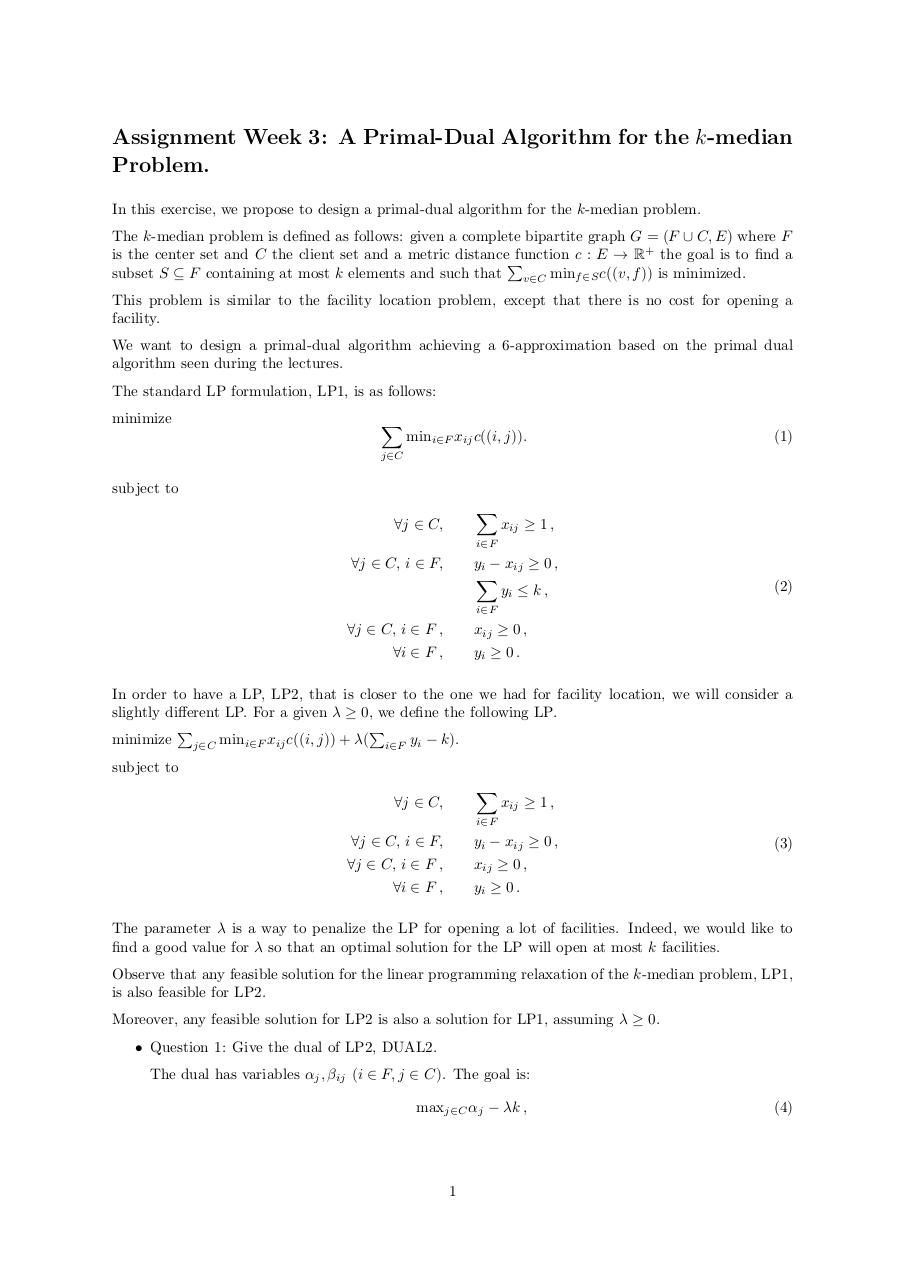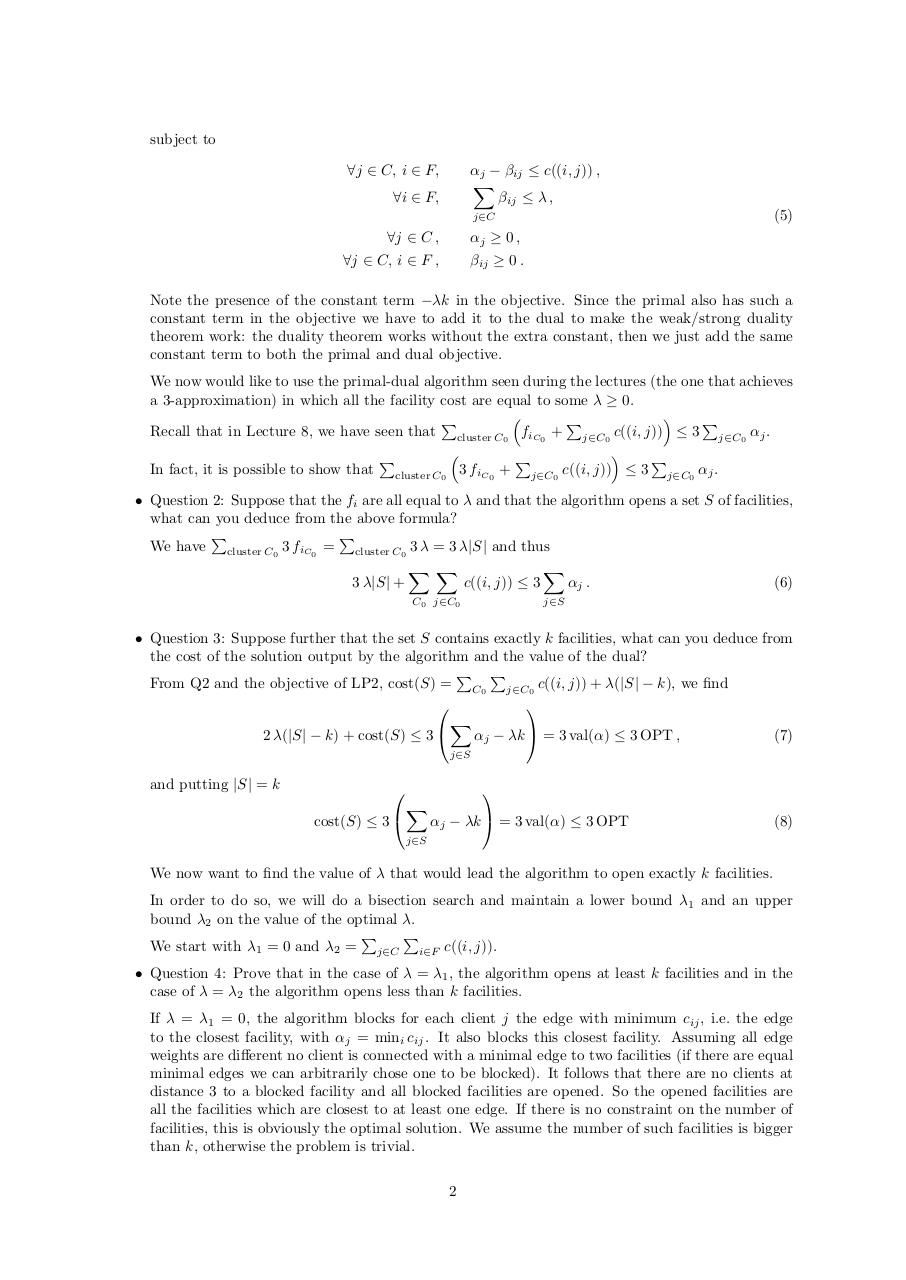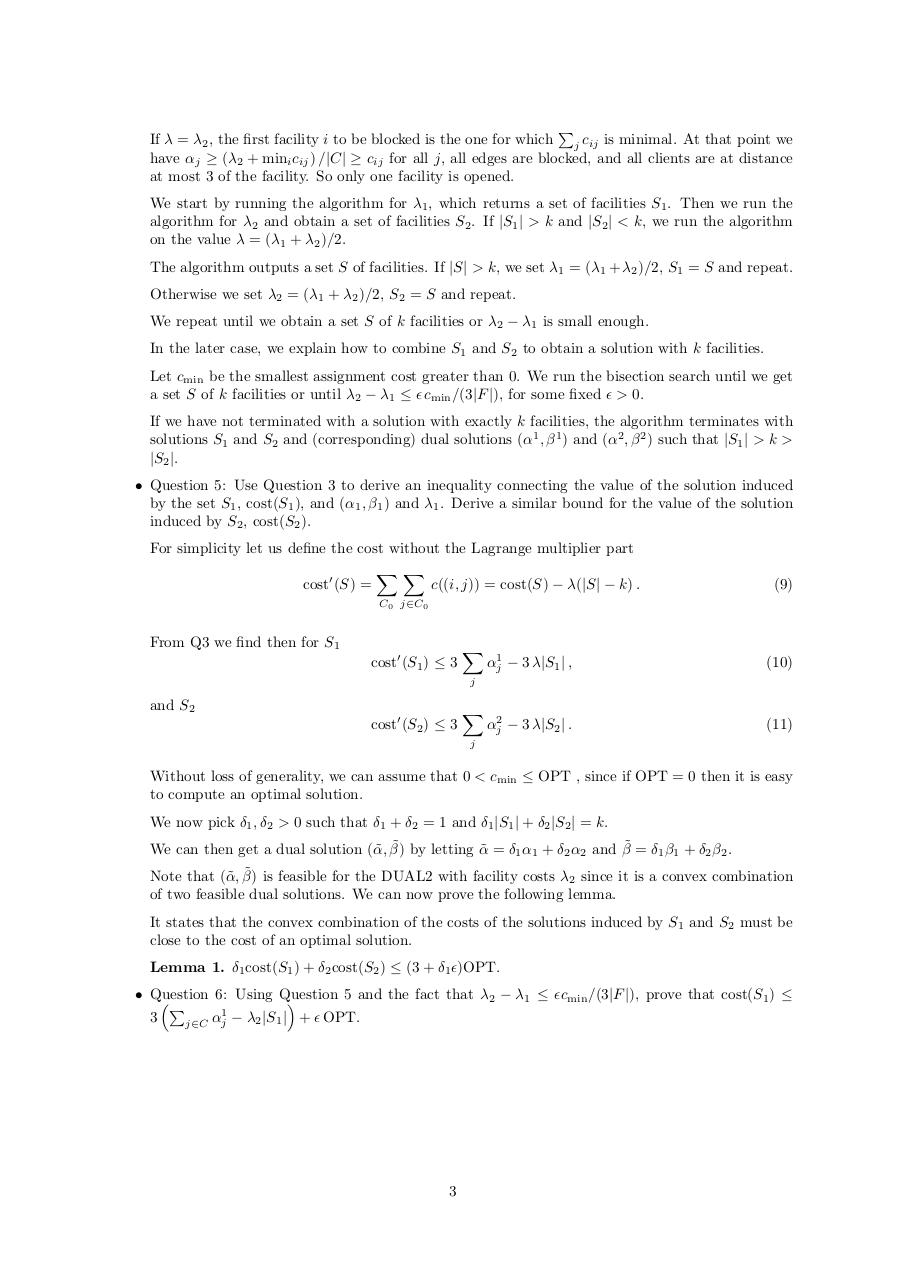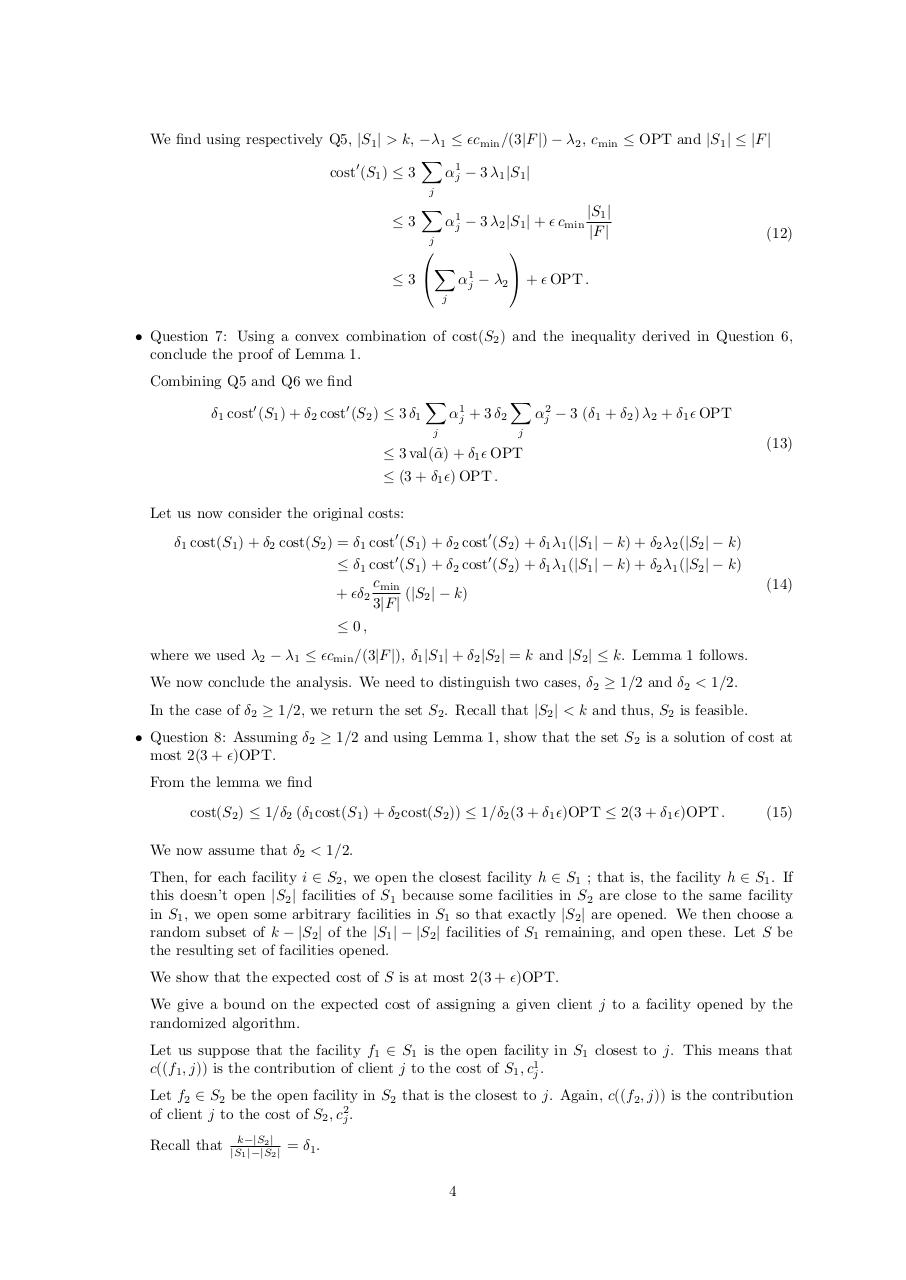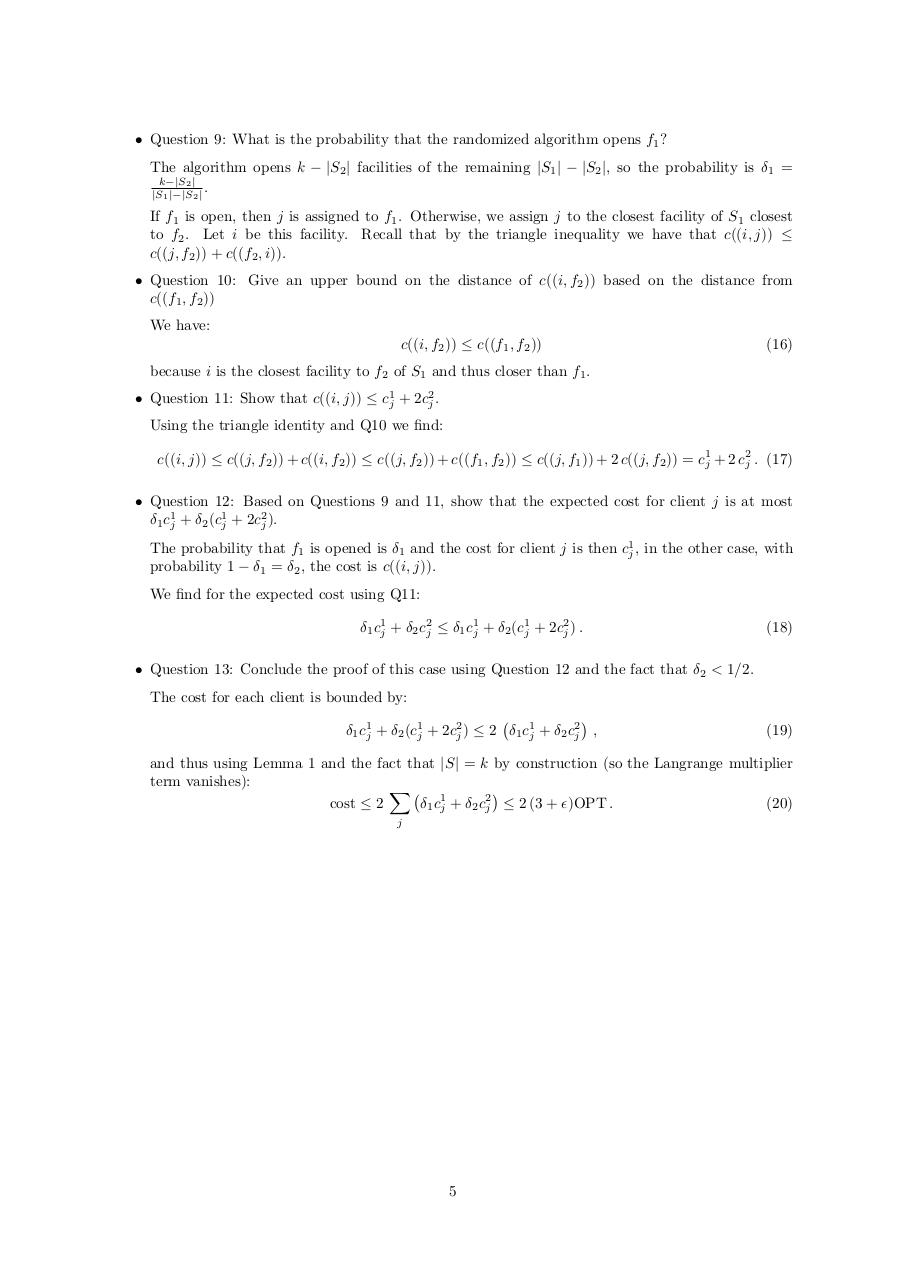#### HTML Code

Copy the following HTML code to share your document on a Website or Blog

#### QR Code### Related keywords